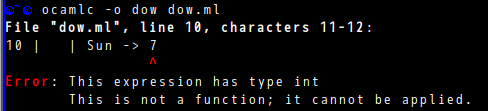# What is wrong with this situation?

I wrote a simple file, like this

``````type weeks = Mon | Tue | Wed | Thu | Fri | Sat | Sun

let day_of_week w =
match w with
| Mon -> 1
| Tue -> 2
| Wed -> 3
| Thu -> 4
| Fri -> 5
| Sat -> 6
| Sun -> 7

print_int (day_of_week Fri)
``````

show me this message when compile itI type the same code in `utop` is working normal.

And i change a little bit of code, it’s also done. like this

``````......
| Sun -> 7;;

.......
``````

Following 2 pics is message on `emacs`

help me plz.
THANKS.

1 Like

also another situation.
a little similar like this

the code

``````let a = "This "
let b = " is "
let c = " a string"

print_string (a ^ b ^ c)
``````

when compile it

``````☯~/code/ocaml☯ ocamlc -o e e.ml
File "e.ml", line 3, characters 8-19:
3 | let c = " a string"
^^^^^^^^^^^
Error: This expression has type string
This is not a function; it cannot be applied.
``````

if i added `;;` on each expression end, then the problem is gone.

what’s wrong with this?

You are correct you need to add a ;; to let the compiler know where the expression ends. Since there is no semicolon, the compiler thinks that you must be trying to call a function given by the previous expression.

``````type weeks = Mon | Tue | Wed | Thu | Fri | Sat | Sun

let day_of_week w =
match w with
| Mon -> 1
| Tue -> 2
| Wed -> 3
| Thu -> 4
| Fri -> 5
| Sat -> 6
| Sun -> 7
;;

print_int (day_of_week Fri)
``````

should be what you want (the whitespace doesn’t matter and is probably what confused you in the original example)

Thanks. I understood it.

But i also have one confuse.
I almost did not see the semicolon in other `OCaml` code file.
This is a Bigest confuse point to me.

Newlines count as white space in OCaml. Thus, the compiler reads

``````let c = " a string"
print_string (a ^ b ^ c)
``````

as

``````let c = " a string" print_string (a ^ b ^ c)
``````

and protests that `"a string"` is not a function.
Rather than using `;;` outside of the REPL, another idiom is to use `let () = ...` :

``````let c = " a string"
let () = print_string (a ^ b ^ c)
``````
2 Likes

THANKS. @octachron

I understood it.
XD